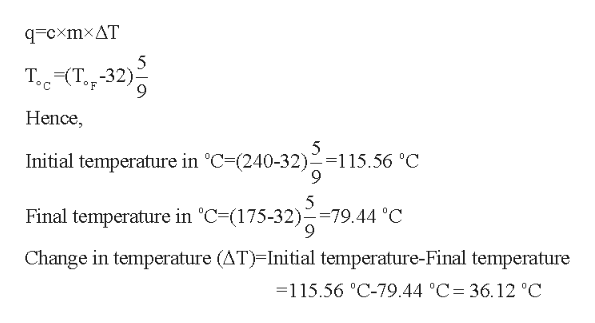# 1. The temperature of the cooling water as it leaves the hot engine of an automobile is 240 °F. After it passesthrough the radiator it has a temperature of 175 °F. Calculate the amount of heat transferred from the engine to thesurroundings by one gallon of water with a specific heat of 4.184 J/g °C

Question
1032 views

1. The temperature of the cooling water as it leaves the hot engine of an automobile is 240 °F. After it passes
through the radiator it has a temperature of 175 °F. Calculate the amount of heat transferred from the engine to the
surroundings by one gallon of water with a specific heat of 4.184 J/g °C

check_circle

Step 1

The amount which is transferred by a given system can be calculated using the following mathematical relation:

Here, q is the heat loss or gain by the system, m is the mass of the substance, delta T is the change (fall or rise) in temperature and c refers to the specific heat which is given to be 4.184 J/g oC for water.

Step 2

The initial and final temperatures in the above problem are given in oF . Hence, firstly they are to be converted into oC as shown below:help_outlineImage Transcriptioncloseq-cxmxAT Т. (Т., -32) F Hence счело335- Initial temperature in °C-(240-32)=115.56 °C 9 5 Final temperature in °C-(175-32)=79.44 °C Change in temperature (AT) Initial temperature-Final temperature - 1 15.56 °C-79.44 °C-36.12 °C fullscreen
Step 3

The volume of water is given to be one gallon.

One gallon is equal to 3.785 L and also 1 L=1000mL.

It is assum...

### Want to see the full answer?

See Solution

#### Want to see this answer and more?

Solutions are written by subject experts who are available 24/7. Questions are typically answered within 1 hour.*

See Solution
*Response times may vary by subject and question.
Tagged in

### Physical Chemistry